DSolve and the General Linear Heterogeneous Case

Mathematica can solve the heterogeneous linear ODE: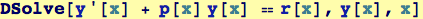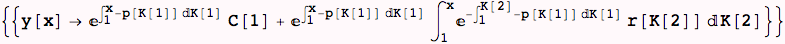Again, we asked Mathematica to solve the most general form of homogeneous linear first-order ODE, and we got a solution in a very general form—two dummy integration variables and one constant of integration. Below, we ask for a specific solution for the heterogeneous case: note that the homogeneous solution can always be added to the specific solution.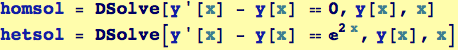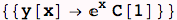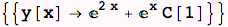Created by Wolfram Mathematica 6.0  (13 November 2007)